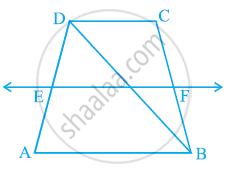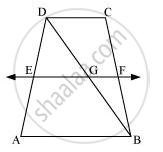# ABCD is a trapezium in which AB || DC, BD is a diagonal and E is the mid - point of AD. A line is drawn through E parallel to AB intersecting BC at F (see the given figure). Show that F is the mid-point of BC. - Mathematics

ABCD is a trapezium in which AB || DC, BD is a diagonal and E is the mid - point of AD. A line is drawn through E parallel to AB intersecting BC at F (see the given figure). Show that F is the mid-point of BC.#### Solution

Let EF intersect DB at G.By converse of mid-point theorem, we know that a line drawn through the mid-point of any side of a triangle and parallel to another side, bisects the third side.

In ΔABD,

EF || AB and E is the mid-point of AD.

Therefore, G will be the mid-point of DB.

As EF || AB and AB || CD,

∴ EF || CD (Two lines parallel to the same line are parallel to each other)

In ΔBCD, GF || CD and G is the mid-point of line BD. Therefore, by using converse of mid-point theorem, F is the mid-point of BC.

Concept: The Mid-point Theorem
Is there an error in this question or solution?

#### APPEARS IN

NCERT Class 9 Maths